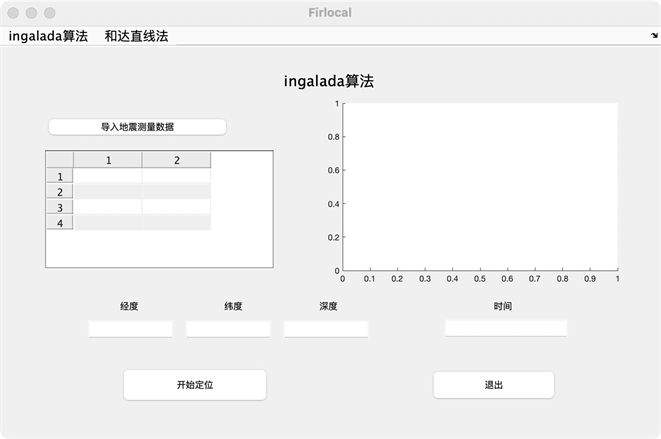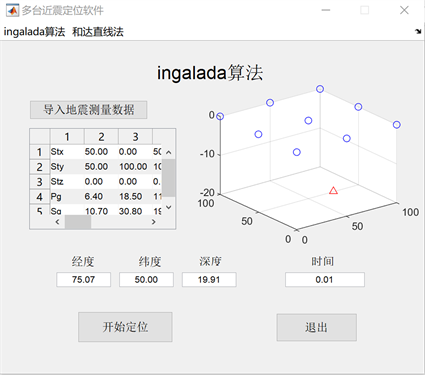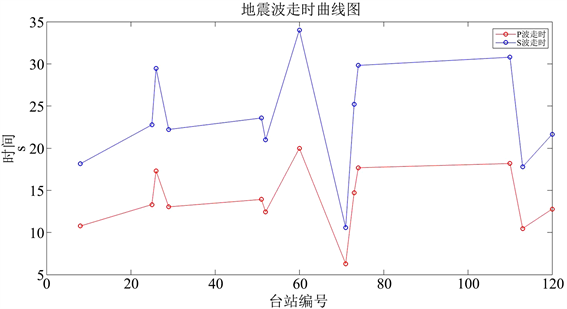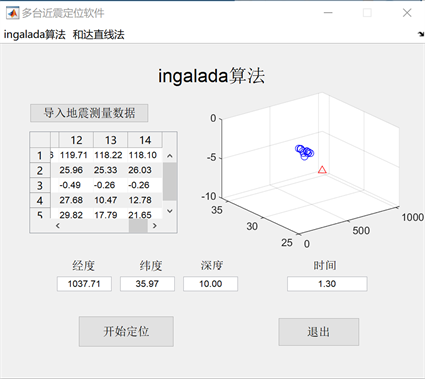#### 期刊菜单

Matlab Visualization of Seismic Positioning

Abstract: Earthquake positioning is one of the most basic research contents in seismology, and it is of great significance for the study of the characteristics of seismic activity, the internal structure of the earth, the geometric structure of the source and other basic issues in seismology. However, direct calculation to obtain the source location information and earthquake occurrence time requires a large amount of calculation and is not easy to implement. In this study, we implemented the INGALADA method positioning through MABLAT to achieve the purpose of determining the location of the earthquake source and the time of occurrence. This earthquake positioning method can be realized by non-professionals. It does not require the operator to have a deep computer and mathematics knowledge. It can directly obtain the actual arrival time of the station P wave, S wave and the position data of the station and import it into the designed GUI display interface. Due to the convenience and intuitiveness of the GUI interface, non-professionals can directly obtain the source location and time of earthquake calculated by the INGALADA algorithm. Seismic positioning can provide some important geographic location information to facilitate the study of geological structure, and is of great significance to the study of earthquake occurrence.

1. 引言

2. 地震定位的原理

${R}_{i}=V\ast \left({P}_{gi}-{S}_{gi}\right)$ (2.2)

${t}_{0}={P}_{gi}-\frac{{R}_{i}}{{v}_{p}}$ (2.3)

3. 地震定位Mablat可视化

1) 震源位置 $\left({x}_{0},{y}_{0},{z}_{0}\right)$ (限制输出数据长度保留两位小数)；

2) 发震时刻(t0) (限制输出数据长度保留两位)。4. 实例测试Figure 4. P and S wave travel time curveTable 1. P, S wave travel time data tableFigure 5. Measured data results display diagram

5. 结论

  田野, 黄汉明, 边银菊, 赵晨杰. 区分天然地震和人工爆炸的可视化方法[J]. 地球科学前沿, 2014, 4(3): 136-146. http://dx.doi.org/10.12677/AG.2014.43017  罗恒之, 罗国富, 任家琪. 银川市周围破坏性地震活动特征及其震感效应[J]. 地球科学前沿, 2019, 9(9): 772-778. https://doi.org/10.12677/AG.2019.99083  天工在线. 中文版MATLAB2018从入门到精通[M]. 北京: 中国水利水电出版社, 2018: 1-530.  傅淑芳, 刘宝诚. 地震学教程[M]. 北京: 地震出版社, 1991.  史鹏程, 王元, 游庆瑜. 利用到时差分布图方法进行近场地表震源定位[J]. 地球物理学报, 2017, 60(8): 3119-3132.  庄昆元, 戴维乐. 虚拟台站法求小范围虚波速度[J]. 地震, 1984(2): 41-45.  赵知军. 宁夏及其邻区虚波速度与震源深度分布[J]. 地震工程学报, 1982, 4(4): 35-40.  万永革. 地震学导论[M]. 北京: 科学出版社, 2017.  万永革. 数字信号处理MATLAB实现[M]. 北京: 科学出版社, 2012: 4-11.  Geiger, L. (1912) Probability Method for the Determination of Earthquake Epicenter from Arrival Time Only. Bulletin of Saint Louis University, 8, 60-71.  高亮, 张守国, 闫峰, 邓阳. Matlab在地震数据绘图中的应用[J]. 防灾减灾学报, 2010, 26(2): 31-35.  廉超, 李胜乐, 董曼, 张卫华. 球面交切法地震定位[J]. 大地测量与地球动力学, 2006, 26(2): 99-103.  王能超. 数值分析简明教程[M]. 第二版. 北京: 高等教育出版社, 2003.  薛慧, 顾玲玲, 谢晓宇. Matlab在地震数据分析中的应用[J]. 商品与质量•科学理论, 2011(4): 173.  张德丰. Matlab数字信号处理与应用[M]. 北京: 清华大学出版社, 2010.